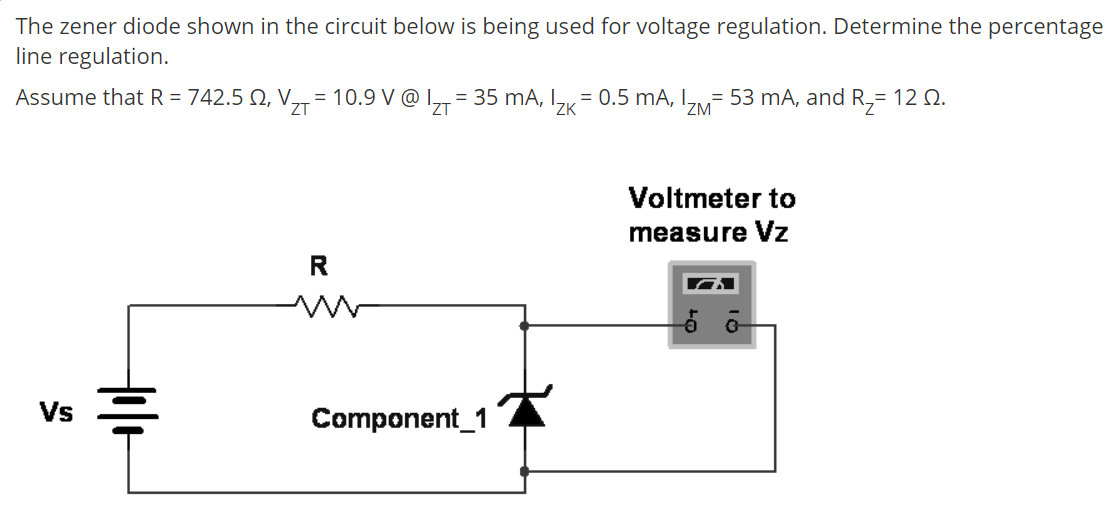# The zener diode shown in the circuit below is being used for voltage regulation. Determine the percentage line regulation. Assume that R = 742.5 N, V,,= 10.9 V @ I,,= 35 mA, I, = 0.5 mA, I= 53 mA, and R,= 12 2. ZT 'ZK 'ZM Voltmeter to measure Vz Vs Component_1

Questionhelp_outlineImage TranscriptioncloseThe zener diode shown in the circuit below is being used for voltage regulation. Determine the percentage line regulation. Assume that R = 742.5 N, V,,= 10.9 V @ I,,= 35 mA, I, = 0.5 mA, I= 53 mA, and R,= 12 2. ZT 'ZK 'ZM Voltmeter to measure Vz Vs Component_1 fullscreen